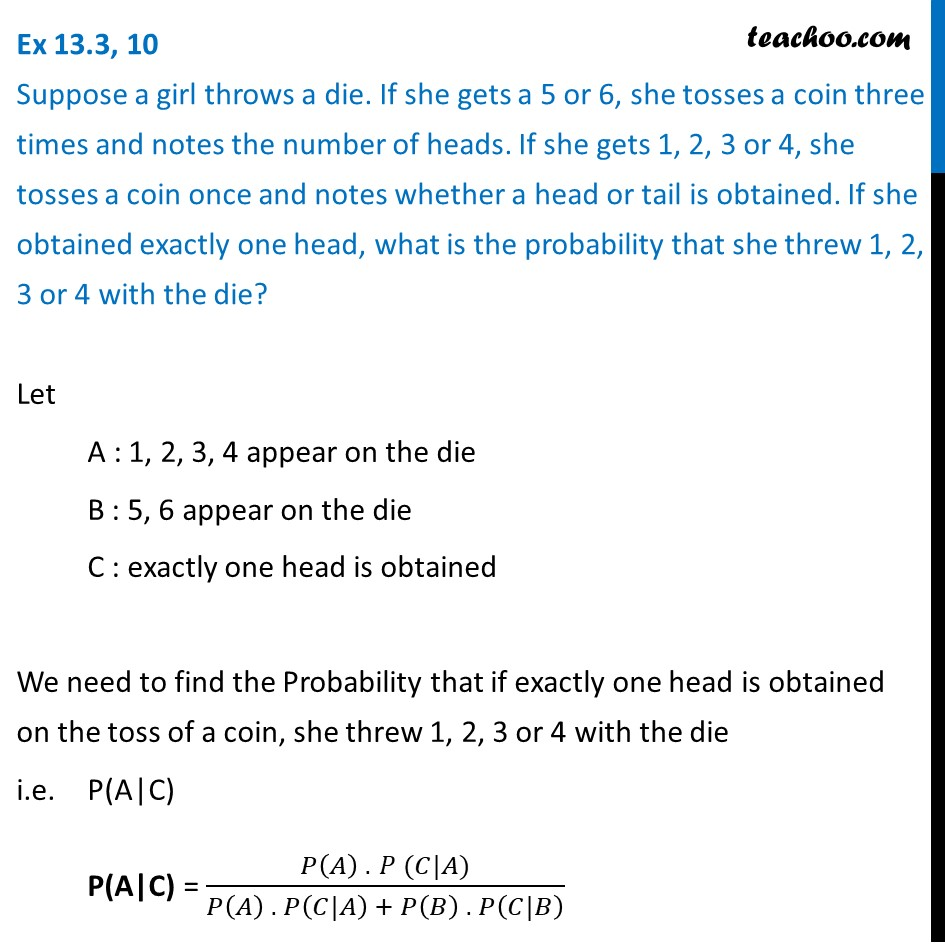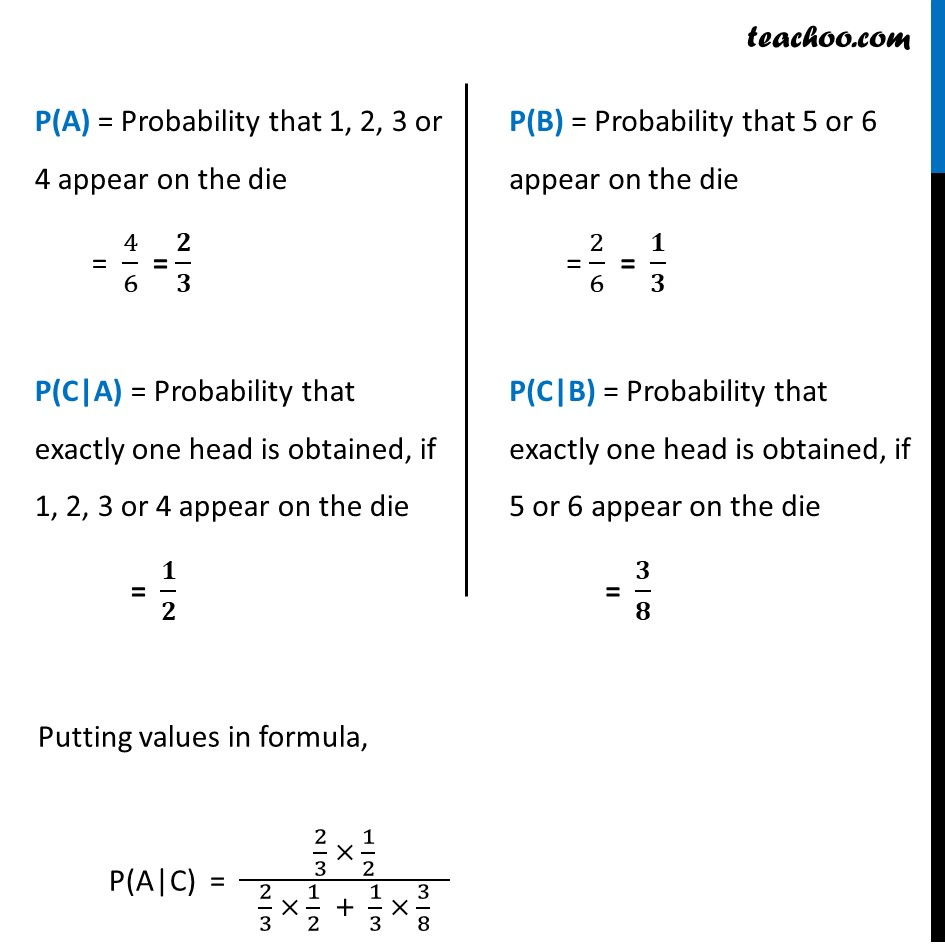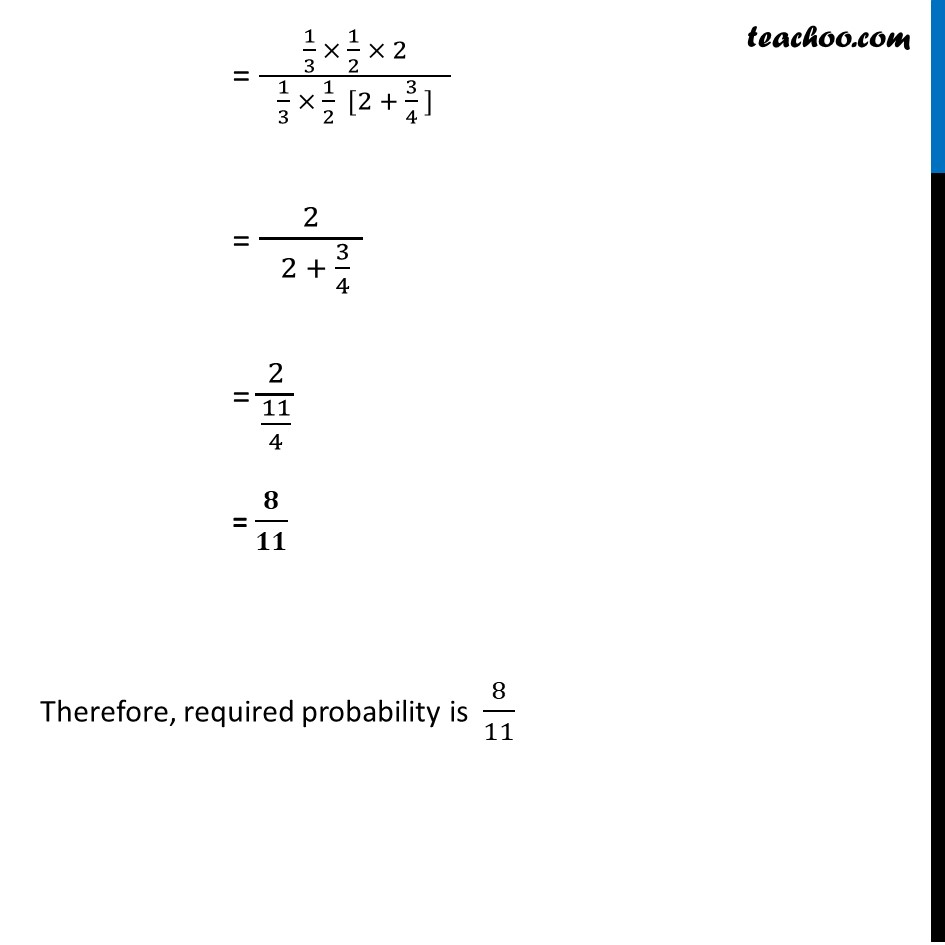Ex 13.3

Chapter 13 Class 12 Probability
Serial order wiseLearn in your speed, with individual attention - Teachoo Maths 1-on-1 Class

### Transcript

Ex 13.3, 10 Suppose a girl throws a die. If she gets a 5 or 6, she tosses a coin three times and notes the number of heads. If she gets 1, 2, 3 or 4, she tosses a coin once and notes whether a head or tail is obtained. If she obtained exactly one head, what is the probability that she threw 1, 2, 3 or 4 with the die?Let A : 1, 2, 3, 4 appear on the die B : 5, 6 appear on the die C : exactly one head is obtained We need to find the Probability that if exactly one head is obtained on the toss of a coin, she threw 1, 2, 3 or 4 with the die i.e. P(A|C) P(A|C) = (𝑃(𝐴) ." " 𝑃(𝐶|𝐴))/(𝑃(𝐴) . 𝑃(𝐶|𝐴) + 𝑃(𝐵) . 𝑃(𝐶|𝐵) ) "P(A)" = Probability that 1, 2, 3 or 4 appear on the die = 4/6 = 𝟐/𝟑 "P(C|A)" = Probability that exactly one head is obtained, if 1, 2, 3 or 4 appear on the die = 𝟏/𝟐 "P(B)" = Probability that 5 or 6 appear on the die = 2/6 = 𝟏/𝟑 "P(C|B)" = Probability that exactly one head is obtained, if 5 or 6 appear on the die = 𝟑/𝟖 = (1/3 × 1/2 × 2)/( 1/3 × 1/2 [2 + 3/4 ] ) = 2/( 2 + 3/4 ) = 2/(11/4) = 𝟖/𝟏𝟏 Therefore, required probability is 8/11